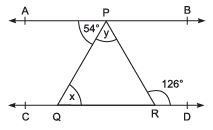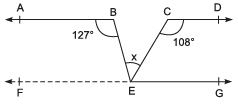# Long Answer Type Questions: Lines & Angles Notes | Study Mathematics (Maths) Class 9 - Class 9

## Class 9: Long Answer Type Questions: Lines & Angles Notes | Study Mathematics (Maths) Class 9 - Class 9

The document Long Answer Type Questions: Lines & Angles Notes | Study Mathematics (Maths) Class 9 - Class 9 is a part of the Class 9 Course Mathematics (Maths) Class 9.
All you need of Class 9 at this link: Class 9

Question 1. In the adjoining  figure, AB || CD. If ∠ APQ = 54° and ∠ PRD = 126°, then find x and y.
Solution:
∵ AB || CD and PQ is a transversal, then interior alternate angles are equal.⇒ ∠ APQ = ∠ PQR
⇒ 54° = x                     [∵ ∠ APQ = 54° (Given)]
Again, AB || CD and PR is a transversal, then ∠ APR = ∠ PRD    [Interior alternate angles]
But ∠ PRD = 126°        [Given]
∴ ∠ APR = 126°
Now, exterior∠ PRD = x + y
⇒ 126° = 54° + y
⇒ y = 126° - 54° = 72°
Thus, x = 54° and y = 72°.

Question 2. In the adjoining figure AB || CD || EG, find the value of x.
Solution:
Through E, let us draw FEG || AB || CD.
Now, since FE || AB and BE is a transversal.
∴ ∠ ABE + ∠ BEF = 180°
[Interior opposite angles]⇒ 127° + ∠ BEF = 180°
⇒ ∠ BEF = 180° ∠ 127° = 53°
Again, EG || CD and CE is a transversal.
∴ ∠ DCE + ∠ CEG = 180°          [Interior opposite angles]
⇒ 108° + ∠ CEG = 180° ⇒ ∠ CEG = 180° - 108° = 72°
Since FEG is a straight line, then
⇒ ∠BEF + ∠BEC + ∠CEG = 180°

[Sum of angles at a point on the same side of a line = 180°]
⇒ 53° + x + 72°
= 180°
⇒ x = 180° - 53° - 72°
= 55°
Thus, the required measure of x = 55°.

The document Long Answer Type Questions: Lines & Angles Notes | Study Mathematics (Maths) Class 9 - Class 9 is a part of the Class 9 Course Mathematics (Maths) Class 9.
All you need of Class 9 at this link: Class 9Use Code STAYHOME200 and get INR 200 additional OFF

## Mathematics (Maths) Class 9

73 videos|351 docs|110 tests

Track your progress, build streaks, highlight & save important lessons and more!

,

,

,

,

,

,

,

,

,

,

,

,

,

,

,

,

,

,

,

,

,

;